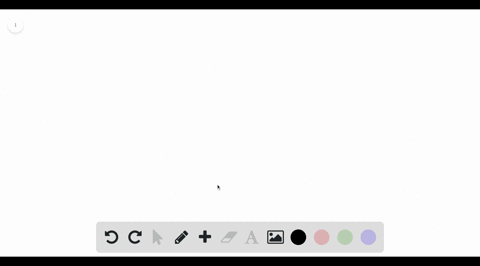Black Friday is Here! Start Your Numerade Subscription for 50% Off!Join Today### Refer to the figure and find the volume generated…

01:04Problem 22

# Refer to the figure and find the volume generated by rotating the given region about the specified line.$\Re_1$ about $BC$

## Discussion

You must be signed in to discuss.

## Video Transcript

in orderto find the volume we know we're integrating along the access parallel to the axis of rotation. We know the axis of rotation is y equals one There, for the outer radius is simply one. The distance from Y equals zero to the oxygen rotation, which we establish is Michael's one, and the in a radius is one minus acts again for the same reasons. Therefore, our volume This pot times the integral from 01 of one squared, minus one minus acts remembered outer minus inner foil and cause bind like terms. Now we can integrate. Use the power rule, increase the expert by one divide by the new exponents. Now we can plug in. We end up with two pi over three.